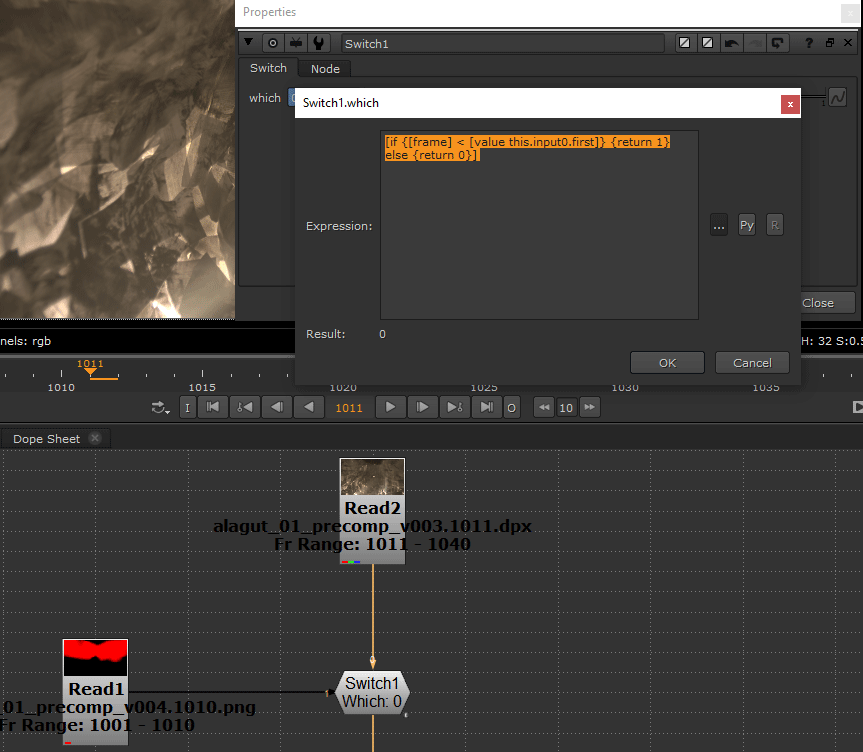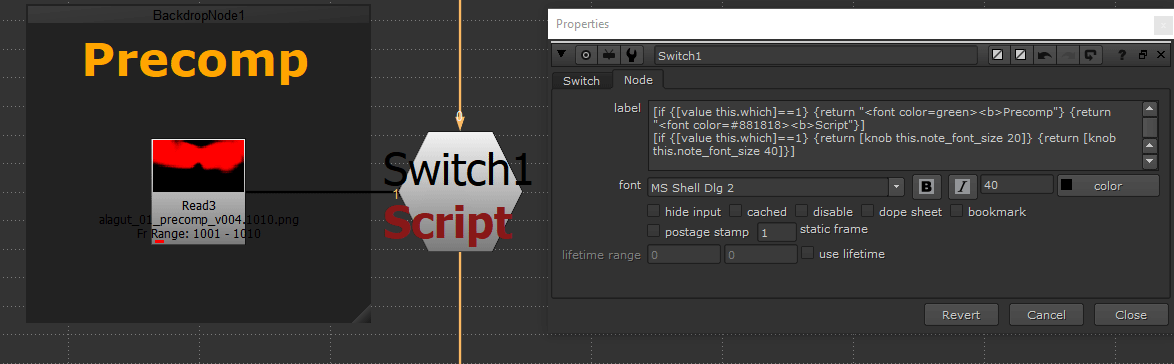## CONDITIONAL TCL EXPRESSIONS IN NUKE

This is a more detailed collection of conditional expressions that can be used in different nodes as parameters of knobs. Here I show different cases that can be especially useful for making templates / gizmos / dynamic scripts or simply want to easily navigate in larger scripts.

For general introduction to expressions, please visit Expressions & TCL Tips.

I will try to explain how to use these snippets for anyone who already familiar with the basics of Nuke.This image shows how if / else statements work in general.

is designed to be a simple but powerful programming language which is ideal to make many things dynamic in Nuke.

Let`s focus on how we can use it by adding parameters that can have multiple outcomes as result.

First with the ways we can write conditions in Nuke.

Only giving a statement a result can be True ( 1 ) or False ( 0 )

condition

e.g.: frame < 1010

When statement is True `if` is the result and `else` when False

condition  ?  if  :  else

e.g.: frame < 1010 ? 50 : 100

Operators

An operator is a symbol that tells the compiler to perform specific

mathematical or logical manipulations.

Arithmetic Operators

+

{value_1  +  value_2  ==  value_3 } ? {return if } : {return else }

e.g.: [if {[value Blur11.size]+[value Blur12.size]==10} {return "500"} {return "10"}]

-

Subtracts second operand from the first

{value_1  -  value_2  ==  value_3 } ? {return if } : {return else }

e.g.: [if {[value Blur11.size]-[value Blur12.size]==10} {return "500"} {return "10"}]

*

Multiplies both operands

{value_1  *  value_2  ==  value_3 } ? {return if } : {return else }

e.g.: [if {[value Blur11.size]*[value Blur12.size]==10} {return "500"} {return "10"}]

/

Divides numerator by de-numerator

{value_1  /  value_2  ==  value_3 } ? {return if } : {return else }

e.g.: [if {[value Blur11.size]/[value Blur12.size]==10} {return "500"} {return "10"}]

%

Modulus Operator and remainder of after an integer division

{value_1  %  value_2  ==  value_3 } ? {return if } : {return else }

e.g.: [if {[value Blur11.size]%[value Blur12.size]==10} {return "500"} {return "10"}]

Relational Operators

==

Checks if the values of two operands are equal or not,

if yes then condition becomes true.

value_1  ==  value_2  ?  if  :  else

e.g.: frame == 1010 ? 50 : 100

!=

Checks if the values of two operands are equal or not,

if values are not equal then condition becomes true.

value_1  !=  value_2  ?  if  :  else

e.g.: frame != 1010 ? 50 : 100

>

Checks if the value of left operand is greater than the value of right operand, if yes then condition becomes true.

value_1  >  value_2  ?  if  :  else

e.g.: frame > 1010 ? 50 : 100

<

Checks if the value of left operand is less than the value of right operand, if yes then condition becomes true.

value_1  <  value_2  ?  if  :  else

e.g.: frame < 1010 ? 50 : 100

>=

Checks if the value of left operand is greater than or equal to the value of right operand, if yes then condition becomes true.

value_1  >=  value_2  ?  if  :  else

e.g.: frame >= 1010 ? 50 : 100

<=

Checks if the value of left operand is less than or equal to the value of right operand, if yes then condition becomes true.

value_1  <=  value_2  ?  if  :  else

e.g.: frame <= 1010 ? 50 : 100

Logical Operators

&&

Called Logical AND operator. If both the operands are non-zero,

then condition becomes true

condition_1  &&  condition_2  ?  if  :  else

e.g.: frame < 1010 && [value mix] == .5 ? 50 : 100

||

Called Logical OR Operator. If any of the two operands is non-zero,

then condition becomes true

condition_1  ||  condition_2  ?  if  :  else

e.g.: frame < 1010 || [value mix] == .5 ? 50 : 100

!

Called Logical NOT Operator. Use to reverses the logical state of its operand.

If a condition is true then Logical NOT operator will make false.

!( condition )

e.g.: !(frame < 1010)

expr

Concatenates args (adding separator spaces between them), evaluates the result as a Tcl expression, and returns the value (more info)

[expr { condition  ?  if  :  else }]

e.g.: [expr {[value Blur9.mix] eq [value Blur8.mix] ? 100 : 20}]

Nuke TCL commands

The following examples shows some of the more commonly used TCL functions in Nuke.

For the whole list visit the Nukepedia Developer Kit.

value

The value tcl command returns the current value of a knob.

[value  knob_name]

e.g.: [value size]==0

frame

Return or set the current frame number.

( in most cases `t` or `x` returns the same value )

frame == value

e.g.: frame == 1010

inrange

Return True ( =1 ) when current frame is in range.

( in most cases `t` or `x` returns the same value as `frame` )

inrange(frame, value_1, value_2)

e.g.: inrange (frame, 1005, 1015)

exists

Returns True ( =1 ) if the named knob or node exists.

[exists  condition]

e.g.: [exists input0]

knob

Returns or sets the entire state of a knob.

This function can be used from any `label` knobs in a way that the condition or/and the result doesn`t affect the node it is written on.

[knob  knob_name  new_value]

e.g.: [if {[value Blur1.size]>10} {return [knob Blur2.size 40]}

{return [knob Blur2.size 20]}]

setkey

Set a value as a keyframe for a knob.

This function can be used from any `label` knobs in a way that the condition or/and the result doesn`t affect the node it is written on.

[setkey  knob_name  frame number  new_value]

e.g.: [if {[value size]==10} {return [setkey Blur20.size 1001 1] &&

[setkey Blur20.size 1010 10]} {return [knob Blur20.size 0]}]

\$gui

Returns False ( =0 ) when Nuke is running and True ( =1 ) when rendering

on the farm. Use ` nuke.executing() ` if need a command

that returns True on local renders too.

\$gui

e.g.: \$gui ? 5 : 10

Useful Snippets

## Using a Switch node`s label to disable inactive nodes in the script.[if {[value this.which]==0} {return [knob VectorBlur1.disable 0] && [knob VectorBlur2.disable 1]} {return [knob VectorBlur1.disable 1] && [knob VectorBlur2.disable 0]}]

## Using a Switch node to automatically change when one of the inputs is out of the frame range.[if {[frame] < [value this.input0.first]} {return 1} else {return 0}]

## Turns True ( =1 ) when input 1 is connected.[exists this.input1]

## Changing label and font size value[if {[value this.which]==1} {return "<font color=green><b>Precomp"} {return "<font color=#881818><b>Script"}]

[if {[value this.which]==1} {return [knob this.note_font_size 20]} {return [knob this.note_font_size 40]}]

## If frame number is greater than 1010 value is 1 else 0

frame > 1010

## Between frame 1010 and 1015 value is 1 else 0

inrange (frame, 1010, 1015)

## 1 if the node's first input is connected else 0

[exists input0]

## 1 if the Input1 node outside the Group is connected else 0

( e.g., for gizmos with `mask` input )

[exists parent.input1]

## If `Read1` node exists returns 1 else 0

## If Input0 has alpha channel returns 1 else 0

[string first ".alpha" [channels [input this 0]]]>-1

## If Blur1 'size' value is 10 returns 1 else 0

[string equal [value Blur1.size] "10"]

## If frame number is lower than 1010 value is 0 else 100.

frame < 1010 ? 0 : 100

## If 'Transform1' translate.x value is 100 the value is 500 else 0

[if {[value Transform1.translate.x]==100} {return "500"} {return "0"}]

## If frame number is 100 the value is 500 else 0

[if {[frame]==100} {return "500"} {return "0"}]

## If 'channels' on 'rgba' return "20" else "10"

[if {[value this.channels] == "rgba" } {return 20} else {return 10}]

Hope you will find it useful!